# Postnikov square

(diff) ← Older revision | Latest revision (diff) | Newer revision → (diff)

A cohomology operation of type, whereandare Abelian groups with a fixed heteromorphism, i.e. a mapping such that the functionis bilinear and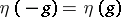. Let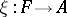be an epimorphism and let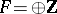be a free Abelian group. The Postnikov square for-cocycles is defined by the formulawhere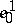is a cochain with coefficients insuch that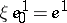. A suspension of a Postnikov square is a Pontryagin square. For a simply-connected space, the Postnikov square for which,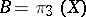andis defined by composition with the Hopf mapping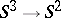is used to classify the mappings of three-dimensional polyhedra into. Postnikov squares were introduced by M.M. Postnikov .

How to Cite This Entry:
Postnikov square. A.F. Kharshiladze (originator), Encyclopedia of Mathematics. URL: http://encyclopediaofmath.org/index.php?title=Postnikov_square&oldid=16646
This text originally appeared in Encyclopedia of Mathematics - ISBN 1402006098